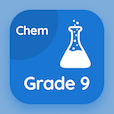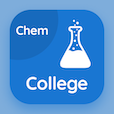Class 9 Courses

Class 9 Chemistry Prep Tests

Class 9 Chemistry Tests

The Book Empirical and molecular formulas Multiple Choice Questions (MCQ Quiz), empirical and molecular formulas quiz answers PDF to learn online courses, 9th grade chemistry tests. Practice Fundamentals of Chemistry Multiple Choice Questions and Answers (MCQs), Empirical and Molecular formulas quiz questions PDF for online school classes. The App Empirical and Molecular formulas MCQ e-Book PDF Download: branches of chemistry, empirical and molecular formulas, ions and free radicals, elements compounds and mixtures test prep for online certificate courses.

The MCQ: The empirical formula for water is PDF, "Empirical and Molecular formulas MCQ" App Download (Free) with co2, ho, h2, and h2o choices for online school classes. Solve fundamentals of chemistry quiz questions, download Google eBook (Free Sample) for distance learning.

## Class 9 Chemistry: Empirical and Molecular formulas MCQs

MCQ: The empirical formula for water is

A) CO2
B) HO
C) H2
D) H2O

MCQ: The molecular formula gives

A) simplest ratio of atoms
B) actual whole number ratio of atoms
C) whole number ratio of atoms
D) natural number ratio of atoms

MCQ: In glucose the simplest ratio between C, H and O is

A) 0.0840625
B) 0.126400463
C) 0.04306713
D) 0.127789352

MCQ: The formula which gives the simplest whole-number ratio of atoms is

A) empirical formula
B) molecular formula
C) chemical formula
D) actual formula

### Practice Tests: Class 9 Chemistry Exam Prep

The Apps: 9th Grade Chemistry Quiz App, College Chemistry MCQs App, and SAT Chemistry MCQ App to download/install for Android & iOS devices. These Apps include complete analytics of real time attempts with interactive assessments. Download Play Store & App Store Apps & Enjoy 100% functionality with subscriptions!